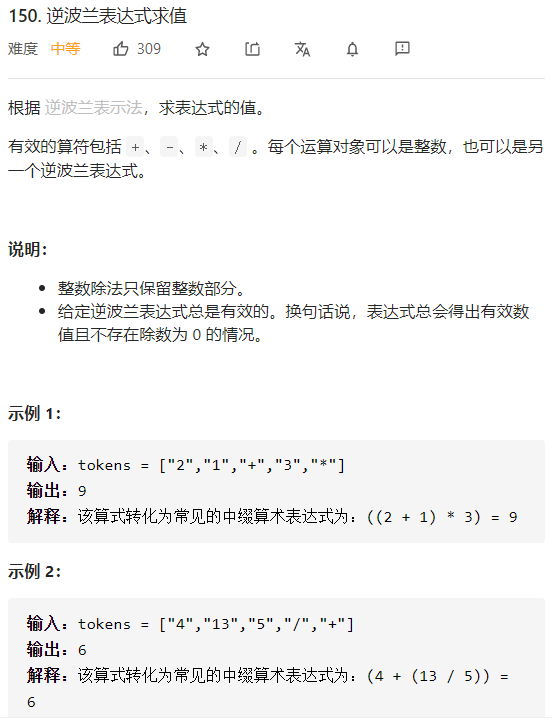# LeetCode每日一题-3.20-150-逆波兰表达式求值

• 时间:
• 浏览:

## 题目描述## 思路 栈

1. 遇到数字，将字符串转换为数字，存入栈中
2. 遇到字符，从栈取出两个数字，第一个作为第二个运算数，第二个作为第一个运算数，将结果存入栈中。

ps:其实也可以使用s - ‘0’。

``````	string a = "3";
int num1 = atoi(a.c_str());
string b = "3";
int num2 = stoi(b);

``````

``````class Solution {
public:
bool isNumber(string s) {
if(!(s == "+" || s == "-" || s == "*" || s == "/")) {
return true;
}
return false;
}
int evalRPN(vector<string>& tokens) {
//存放数字的堆栈
stack<int> s;
//这里用for(string c : tokens)也是对的
for(const string& c : tokens) {
if(isNumber(c)) {
s.push(stoi(c));
} else {
int tmp;
int b = s.top();
s.pop();
int a = s.top();
s.pop();
switch(c) {
case '+':
tmp = a + b;
break;
case '-':
tmp = a - b;
break;
case '*':
tmp = a * b;
break;
default:
tmp = a / b;
}
s.push(tmp);
}
}
return s.top();
}
};
``````